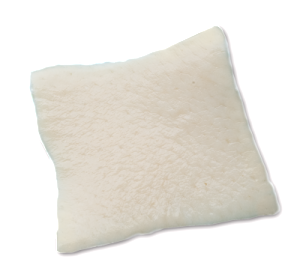# Acellular Dermis – PSMatrix

Acellular Dermal Matrix (ADM) is donated human skin that has gone through a variety of processes that removes the cells from the graft that ultimately could cause tissue rejection. In addition to the removal of cells, ADM’s go through extensive testing to ensure donor viability and minimal risk of disease transmission. PSMatrix is also sterilized using low dose gamma irradiation that gives it a Sterility Assurance Level of 10-6 .
Product Code Description Measurements
OPT-M22 PSMatrix 0.5 Extra Small W=2cm L=2cm T=0.5mm
OPT-M44S PSMatrix 0.5 Small W=5cm L=4cm T=0.5mm
OPT-M44M PSMatrix 0.75 Small W=5cm L=4cm T=0.75mm
OPT-M46S PSMatrix 0.5 Medium W=7cm L=5cm T=0.5-1mm
OPT-M46M PSMatrix 0.75 Medium W=7cm L=5cm T=1-2mm
OPT-M510S PSMatrix 0.75 Large W=5cm L=10cm T=0.5-1mm
OPT-M510M PSMatrix 1.5 Large W=5cm L=10cm T=1.0-2mm
OPT-M816 PSMatrix 1.5 XL W=8cm L=16cm T=1.5mm
OPT-M1620 PSMatrix 1.5 XXL W=16cm L=20cm T=1.5mm